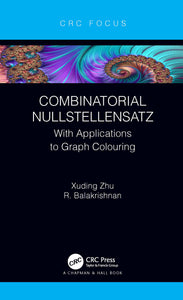# Combinatorial Nullstellensatz : With Applications to Graph Colouring

Regular price \$22.95 \$22.95 Sale

Author(s): Xuding Zhu, R. Balakrishnan

Combinatorial Nullstellensatz is a novel theorem in algebra introduced by Noga Alon to tackle combinatorial problems in diverse areas of mathematics. This book focuses on the applications of this theorem to graph colouring. A key step in the applications of Combinatorial Nullstellensatz is to show that the coefficient of a certain monomial in the expansion of a polynomial is nonzero. The major part of the book concentrates on three methods for calculating the coefficients:

1. Alon-Tarsi orientation: The task is to show that a graph has an orientation with given maximum out-degree and for which the number of even Eulerian sub-digraphs is different from the number of odd Eulerian sub-digraphs. In particular, this method is used to show that a graph whose edge set decomposes into a Hamilton cycle and vertex-disjoint triangles is 3-choosable, and that every planar graph has a matching whose deletion results in a 4-choosable graph.
2. Interpolation formula for the coefficient: This method is in particular used to show that toroidal grids of even order are 3-choosable, r-edge colourable r-regular planar graphs are r-edge choosable, and complete graphs of order p+1, where p is a prime, are p-edge choosable.
3. Coefficients as the permanents of matrices: This method is in particular used in the study of the list version of vertex-edge weighting and to show that every graph is (2,3)-choosable.

It is suited as a reference book for a graduate course in mathematics.

Review(s):

EAN: 9781000426687